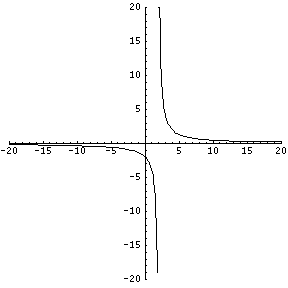## Pre-Calculus Tutorial

#### IntroYou can tell the function graphed above has a vertical asymptote at x=2 as the graph on the left and right sides of x=2 approach negative infinity and positive infinity, respectively. However, how do we determine the vertical asymptote(s) of a rational function without a graph?

In order to find a vertical asymptote of a rational function, simply set the value of the denominator equal to zero, and solve for all x values. At these x-values, the function is dividing by zero, which gives an undefined result. Close to x=2, the function is dividing by numbers extremely close to zero, i.e. 0.00001. This results in extremely large values that approach infinity, or possibly negative infinity. Let’s try solving a problem.

#### Sample Problem

What are the vertical asymptotes of the following function?,,#### Solution

First, we set the denominator equal to zero.Then, we solve the equation for x. This may require use of the quadratic equation, but more likely just some quick factoring. In this case, the function may be factored in to (x+3) and (x-4).Therefore, the polynomial in the denominator has roots at x=-3 and x=4. Therefore, the function has vertical asymptotes at x=-3 and x=4, as these are the values at which the bottom is divided by zero.